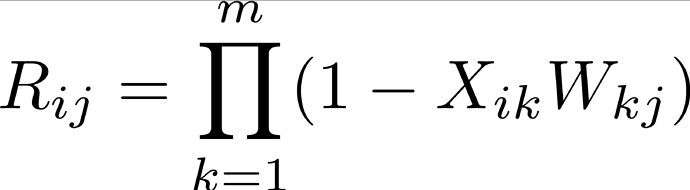# How can I implement this calculation without large memory usage?

I have two tensors, X and W, and their shapes are (n, m) and (m, l), respectively. I want to get a new tensor R that. My current solution is using `torch.prod(1 - X.unsqueeze(-1) * W, dim=1)` . However, it will generate a tensor in the shape of (n, m, l) to store intermediate results. This (n, m, l) tensor could be too large to store in my GPU. Is there any way to avoid this large intermediate result tensor and can still keep the speed? For example, the calculation to get R is very similar to matrix multiplication, but the matrix multiplication costs much less memory.